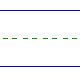Name: ______________________________________________ Date: _____________

Multiplication

Instructions: Multiply.

 6 x 2 =9 x 5 =2 x 2 =0 x 7 =3 x 3 =3 x 2 =2 x 0 =4 x 3 =4 x 7 =2 x 9 =5 x 2 =3 x 9 =6 x 1 =6 x 5 =4 x 0 =1 x 7 =5 x 0 =0 x 0 =